Optical Rotation

Let us first examine the case where left and right circularly polarized light are affected differently by the refractive index but not by the extinction coefficient. The real part of the complex refractive index (i.e. the refractive index) is defined as n = c / vP where c is the speed of light in empty space. If while traveling within a material, left and right circularly polarized light propagate with a different phase velocity, then after emerging from the medium, the arrows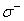and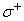would complete different parts of the circle. However, since there is no difference in absorption, both arrows would exhibit equal amplitudes. The resulting polarization is schematized in figure 2, where, as you can see, at time t = 0,has not completed its circular motion and the resulting linear polarization vector (blue arrow) appears to be rotated from its original position by an angle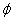. This is called optical rotation or circular birefringence.

Fig. 2 Linearly polarized light as a combination of left () and right () circularly polarized light in the case where the latter two propagate with different phase velocities in the material, (i.e. the medium has a different refractive index forand) but experience similar extinction coefficients.

It is very straightforward to evaluatein terms of n- and n+ the respective refractive indices forand. Circularly polarized light is described by a set of two linearly polarized electromagnetic waves. Thus for left and right circular polarizations: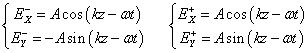(Eq.2.1)

which can be rewritten as:  (since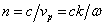)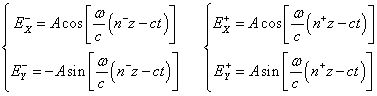(Eq.2.2)

and we can then further rewrite: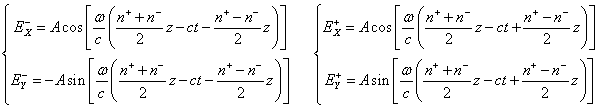(Eq.2.3)

If we then define: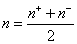(Eq.2.4)

equations 2.3 become: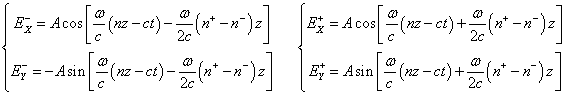(Eq.2.5)

Recognizing the opportunity to apply the formula for sin and cos sum and difference: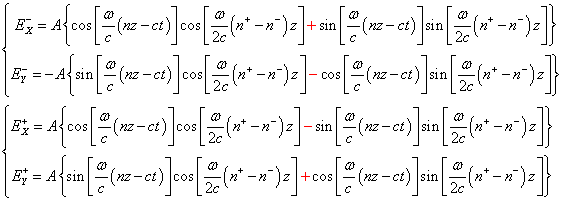(Eq.2.6)

Now we can combine the x-components and the y-components of these circular polarizations in order to evaluate the resulting linear polarization: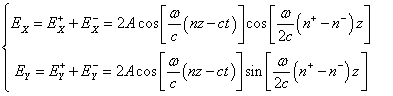(Eq.2.7)

Equations 2.7 represent a linearly polarized wave having an angle of polarizationwith respect to the x-axis such as:(Eq.2.8)

where you can recognize: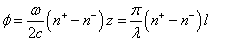(Eq.2.9)

with l being the length of the material. Note that this angle is in radians and must be multiplied by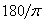in order to obtain the value in degrees.